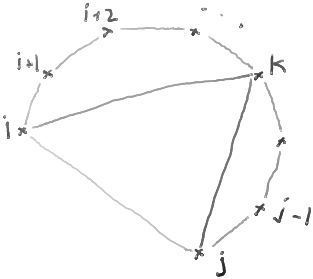MinWtTriangulationByDP

ClassS04CS141 | recent changes | Preferences

Difference (from prior major revision) (no other diffs)

Changed: 35c35

Minimum weight triangulation

There are n points {1,2,...,n} arranged on a circle in the plane. You are given n and a matrix of costs cost[i,j], where cost[i,j] is the cost of the line segment connecting point i to point j.

A triangulation T is a collection of line segments connecting the points such that

• none of the line segments in T cross
• the regions defined by the line segments in T are triangles (in other words, no other line segment can be added to T without crossing some line segment in T).

The total cost of the triangulation T is the total cost of the line segments in T.

The goal is to find a triangulation of minimum total cost.

claim: Each triangulation of {1,2,...,n} contains a triangle {1,k,n} for some k in {2,3,...,n-1}

claim: Each triangulation of {1,2,...,n} is formed by the triangle {1,k,n} for some k together with a triangulation of {1,2,...,k} and a triangulation of {k,k+1,...,n}.

More generally:

claim: Each triangulation of {i,i+1,...,j} contains a triangle {i,k,j} for some k in {i+1,i+2,...,j-1}

claim: Each triangulation of {i,i+1,...,j} is formed by the triangle {i,k,j} for some k together with a triangulation of {i,2,...,k} and a triangulation of {k,k+1,...,j}.

picture:?

Counting the number of triangles in a triangulation

Using the above claim, we can give a recurrence for the maximum number of triangles in a triangulation of n points:

N(n) = maxk=2,..,n-1 1+N(k)+N(n-k+1)
N(2) = 0

This gives N(n) = n-2 (prove by induction).

Counting the number of triangulations

We can also give a recurrence for the number of triangulations of n points:

N(n) = k=2,..,n-1 N(k)*N(n-k+1)
N(2) = 1

Finding the minimum cost of any triangulation

claim: The minimum-cost triangulation of {1,2,...,n} is composed of the segment {1,n} together with a minimum-cost triangulation of {1,2,..,k} and a minimum-cost triangulation of {k,k+1,...,n}.

Define T[i,j] = the minimum cost to triangulate {i,i+1,...,j-1,j}.

Then

T[i,i+1] = cost[i,i+1] (as a degenerate case)
T[i,i+2] = cost[i,i+1]+cost[i+1,i+2]+cost[i,i+2] and
T[i,j] = min { cost[i,j] + T[i,k] + T[k,j] : k = i+1,i+2,...,

This recurrence gives an O(n3) algorithm:

1.  min_cost_triangulation(i, j, cost, cache)
2.    if (j == i+1) return cost[i,i+1]
3.    if (j == i+2) return cost[i,i+1]+cost[i+1,i+2]+cost[i,i+2]
4.    if ! cache.exists(i,j)
5.        cache[i,j] = cost[i,j] + min { T[i,k] + T[k,j] : k = i+1,i+2,...j-1 }
6.    return cache[i,j]

The final answer is given by min_cost_triangulation(1, n, cost, cache).

References

ClassS04CS141 | recent changes | Preferences